# Voltage in Series Circuits (Sources, Formula & How To Add)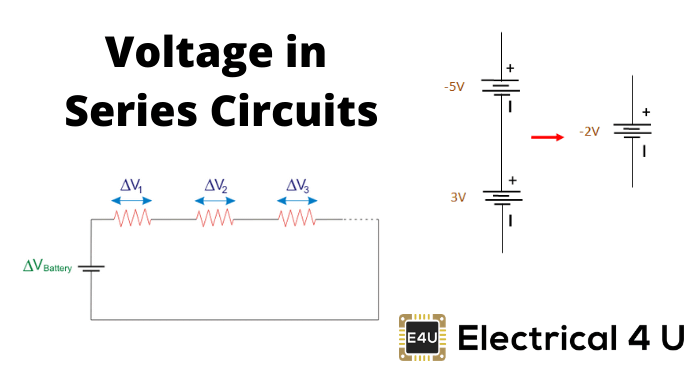## What are Voltages in Series?

A series circuit or series connection refers to when two or more electrical components are linked together in a chain-like an arrangement within a circuit. In this kind of circuit, there is only a single way for charge to pass through the circuit. The potential variation in charge across two points in an electrical circuit is known as voltage. In this article, we will discuss in detail voltages in a series circuit.

The battery of a circuit provides energy for the charge to pass through the battery and to create a potential difference among the ends of the external circuit. Now, if we assume a cell of 2 volts, it will create a potential difference of 2 volts across the external circuit.

The electric potential value at the positive terminal is 2 volts greater than the negative terminal. So, when charge flows from the positive to the negative terminal, it causes a loss of 2 volts in electrical potential.

This is termed as voltage drop. This happens when the electrical energy of the charge is converted into some other forms (mechanical, heat, light etc) while passing through the components (resistors or load) in the circuit.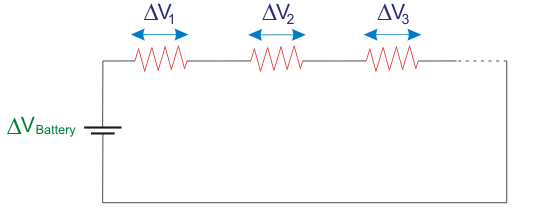If we consider a circuit with more than one resistor connected in series and are powered with a 2V cell, the total loss of electrical potential is 2V. That is, there will be a certain voltage drop in each connected resistor. But we can see that the sum of the voltage drop of all the components will be 2V which is equivalent to the voltage rating of the power source.

Mathematically, we can express it as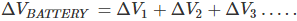By using Ohm’s law the individual voltage drops can be calculated as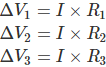Now, we can assume a series circuit comprises of 3 resistors and powered by a 9V energy source. Here, we are going to find out the potential difference at a different location during the passage of current throughout the series circuit.

The locations are marked in red color in the circuit below. We know that current passes in the direction from the positive terminal towards the negative terminal of the source. The negative sign of the voltage or potential difference represents the loss in potential due to the resistor.

The electrical potential difference of different points in the circuit can be represented with the help of a diagram called the electric potential diagram that is shown below.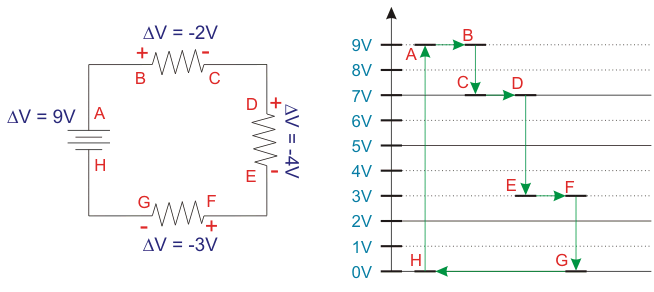In this example, the electrical potential at A = 9V since it is the higher potential terminal. The electrical potential at H = 0V since it is the negative terminal. When the current passes through the 9V power source, the charge gains 9V of electrical potential, which is from H to A. While the current passes throughout the external circuit, the charge loses this 9V completely.

Here, this happens in three steps. There will be drop-in voltage when the current passes through the resistors but no voltage drop occurs when the passage is through the mere wire. So, we can see that between the points AB, CD, EF and GH; there is no voltage drop. But between points B and C, the voltage drop is 2V.

That is the source voltage 9V becomes 7V. Next, between the points D and E, the voltage drop is 4V. At this point, the voltage 7V becomes 3V. At last, between the points F and G, the voltage drop is 3V. At this point, the voltage 3V becomes 0V.

The portion of the circuit between the points G and H, there is no energy for the charge. So, it desires an energy boost for the passage through the external circuit again. This is provided by the power source as the charge passes from H to A.

The several voltage sources in series can be replaced by a single voltage source by taking the sum total of all the voltage sources. But we have to consider the polarity as shown below.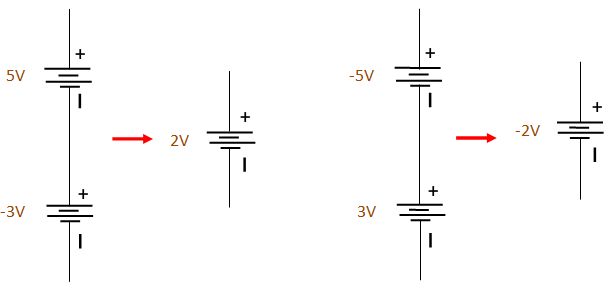## AC Voltage Sources in Series

In the case of AC voltage sources in series, the voltage sources can be added or combined together to form a single source provided that the angular frequency (ω) of the connected sources are identical. If the AC voltage sources connected in series are of different angular frequencies, it can be added together provided the current through the connected sources is the same.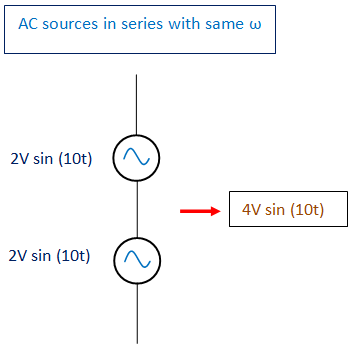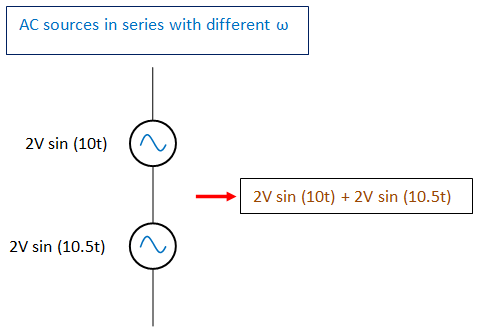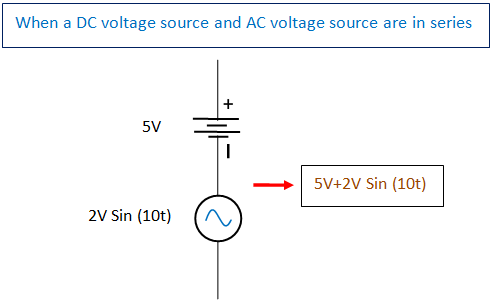## Application of Voltage in Series Circuits

The application of voltages in series circuits include:

• Voltage divider.
• Fire alarm battery.
• Batteries in remote, toys etc.
• Lighting purposes in a train, Christmas tree etc.

Want To Learn Faster? 🎓
Get electrical articles delivered to your inbox every week.
No credit card required—it’s 100% free.